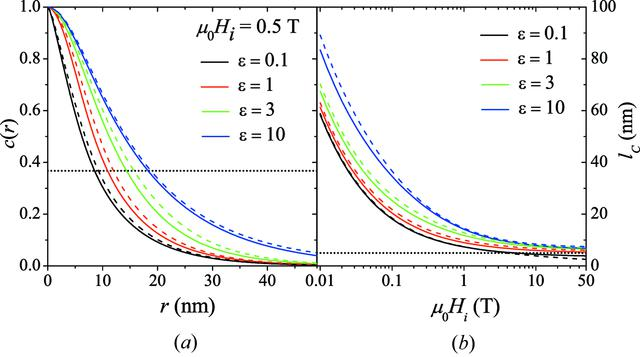disable zoom     view article Figure 8 Effect of particle form factor on the correlation function and correlation length. (a) c(r) at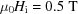and for several particle form factors. Solid lines: form factor of ellipsoid of revolution (R = 5  nm) with ∊ decreasing from top to bottom (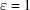corresponds to the sphere form factor). Dashed lines: cylinder form factor with R = 5  nm and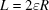[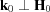;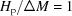; S(q) = 1]. Dotted horizontal line: c(r) = exp(-1). (b) Corresponding lC(Hi) (log–linear scale). Dotted horizontal line: lC = R = 5  nm.JOURNAL OFAPPLIEDCRYSTALLOGRAPHY
ISSN: 1600-5767
Volume 48| Part 5| October 2015| Pages 1437-1450